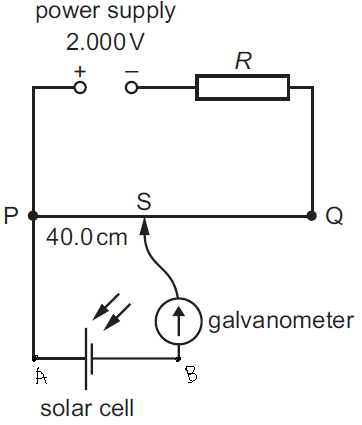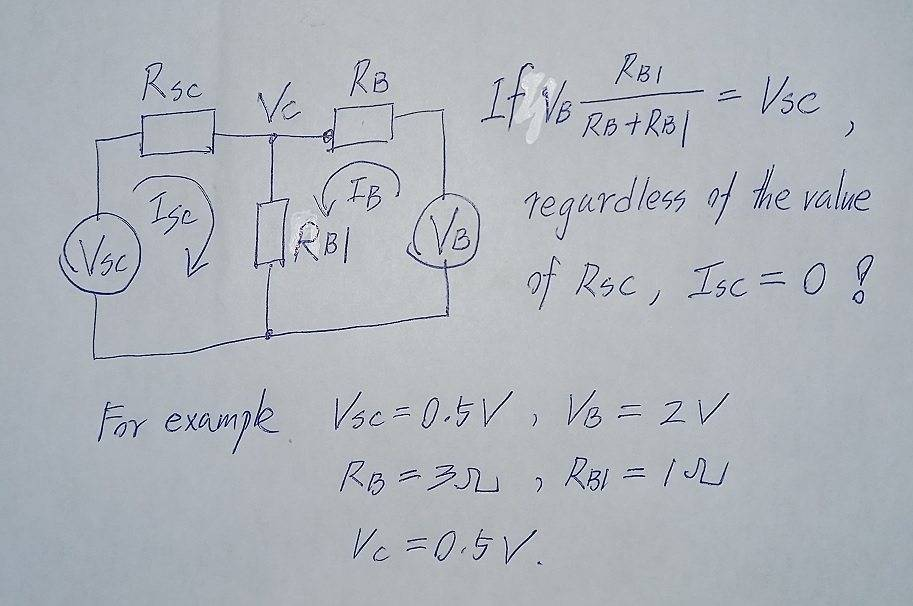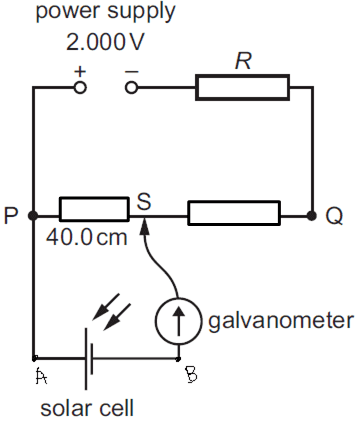# Question about potential and current in potentiometer circuit

• songoku
In summary, Kirchhoff's laws still apply to a circuit loop, but they are not applied equally across a voltage source (PS). If the potentiometer is not balanced, current will flow from P to A and from B to S.

#### songokuLet say S is the balanced point so current flowing through galvanometer is zero. This is because the potential difference across PS is the same as potential difference across solar cell so no potential difference means no current flowing.

My questions:
1) If I want to compare the potential at a point, is it correct to say V at P = V at A and V at S = V at B?

2) There is potential difference between point A and B (which is the same as the emf of solar cell) so why no current flows from B to A or A to B?

3) If point S is moved to the right (towards Q), then potential difference across PS > emf of solar cell then current will flow through galvanometer. How to determine whether the current will flow from A to B or B to A? And I think PS and AB are parallel and in parallel connection the potential difference will be the same but VPS ≠ VAB, what am I missing here?

Thanks

1) Correct
2) There is no potential difference from A in any direction, nor from B
3) PS potential difference will be greater than AB potential difference, so current will flow from P to A and from B to S.
songoku said:
And I think PS and AB are parallel and in parallel connection the potential difference will be the same but VPS ≠ VAB, what am I missing here?
Ergo they are not parallel ! There is resistance PS beetween teh endds on one side and the solar cell between the ends on the other.

They carry the same current, so at best they are in series.

BvU said:
1) Correct
If now the point S is moved to the right towards Q, is it still correct to say V at P = V at A? If yes, then it means no potential difference between P and A so why current can flow from P to A?

2) There is no potential difference from A in any direction, nor from B
I assume B has zero potential (grounded) and let say the solar cell has emf of 0.5 V. Is it correct to say potential at A is 0.5 V and at B is zero? If yes, why no current flows even though there is potential difference? Or the thing I write is completely different matter compared to the picture?

Ergo they are not parallel ! There is resistance PS beetween teh endds on one side and the solar cell between the ends on the other.

They carry the same current, so at best they are in series.
Sorry I don't understand this part. You mean when the potentiometer is not balanced, the current flowing through solar cell will be the same as the current flowing through PS?

Thanks

To answer (2), Kirchhoff's laws apply to circuit loops, and do not apply simply across a voltage source. For a single resistor, you do have Ohm's law, but Ohm's law does not apply across a voltage source.

For the first question, in an ideal conductor (wire), you can get current flowing in the wire, even though the wire has essentially the same voltage everywhere. The resistance though not precisely zero, is often negligible.

Last edited:
songoku said:
2) There is potential difference between point A and B (which is the same as the emf of solar cell) so why no current flows from B to A or A to B?

I think it should be similar to this situation.Last edited:
@songoku : is it clear to you now ?

BvU said:
@songoku : is it clear to you now ?
I still have something I don't understand.

songoku said:
Sorry I don't understand this part. You mean when the potentiometer is not balanced, the current flowing through solar cell will be the same as the current flowing through PS?

Thanks

songoku said:
If now the point S is moved to the right towards Q, is it still correct to say V at P = V at A? If yes, then it means no potential difference between P and A so why current can flow from P to A?
Maybe it is somwhat confusing that in the circuit diagram PA looks the same as PSQ. They are intended to be different:
PA is supposed to be an ideal conductor (zero resistance, potential the same at both ends and nevertheless a current can flow, the magnitude of which is determined elsewhere. Remember V = IR).
PQ is supposed to be a wire with resistance, so that there is a voltage drop.
If The wire length is LPQ and the resistance is RPQ, then
RPS / RPQ = LPS / LPQ.​
And if the galvanometer shows zero:
VPS / VPQ = LPS / LPQ
the voltage drop is proportional to the length L. In a picture:So the idea is that if ##\ L_{PS} = 40 ## cm and ##\ L_{PQ}\ = 100 ## cm (are you sure that wasn't in the exercise statement ?) that -- if the galvanometer shows zero -- then ##V_{PS} = 40/100 * 2.00 ## Volt.

This exposes a weakness in the exercise: nothing is said about R. If the idea is to determine the voltage that the solar cell produces by making use of the $$V_{PS} = V_{PQ} * {L_{PS}\over L_{PQ} }$$ and ##\ V_{PS} = V_{AB}\ ## when the galvanometer shows zero, then still ##\ V_{PQ}\ ## has to be known.​
Either through a measurement or by allowing the approximation ##\ V_{PQ}\ \approx 2.000 ## Volt. Such an approximation can only be made if ##\ R << R_{PQ}\ ##.​
The composer of the exercise should have left out R altogether: its function is current limitation, but the 2.000 V and the 40.0 suggest an accuracy in the milliVolt range, so the voltage dop over R should be less than a mV. If e.g. ##\ R_{PQ} = 10 \Omega\ ## that means ##R < 0.01 \Omega\ ## and therefore useless as current limiter.​

I assume B has zero potential (grounded)
You can certainly do that. But it serves little purpose: everything here is about voltage differences. It may even be confusing, because it's not a constant reference point if S moves.

and let say the solar cell has emf of 0.5 V. Is it correct to say potential at A is 0.5 V and at B is zero? If yes, why no current flows even though there is potential difference? Or the thing I write is completely different matter compared to the picture?
Yes: ##\ V_A = 0.5 ## V and B was defined to be zero.
No current flows in AB because ##\ V_{PS} ## is also 0.5 V.

Sorry I don't understand this part. You mean when the potentiometer is not balanced, the current flowing through solar cell will be the same as the current flowing through PS?
No.

I resume:

BvU said:
3) PS potential difference will be greater than AB potential difference, so current will flow from P to A and from B to S.
songoku said:
And I think PS and AB are parallel and in parallel connection the potential difference will be the same but VPS ≠ VAB, what am I missing here?
Ergo they are not parallel ! There is resistance PS between the ends on one side and the solar cell between the ends on the other.

They carry the same current, so at best they are in series.

I think I made a mess of that, sorry. Strike the last sentences -- must have been the lack of coffeeBut then "3) PS potential difference will be greater than AB potential difference, so current will flow from P to A and from B to S." does not make much sense either !

Let me try again -- altough it looks much the same ...

If S is moved away to the right from the balanced point , ## \ V_{PS} \ ## will be greater than the solar cell voltage and current will flow from P to S through the cell, so from A to B.

One thing sure to happen is that the current trough AB will increase the voltage drop over RS, thereby reducing ## \ V_{PS} \ ##
In addition, the voltage drop ## \ V_{AB} \ ## may increase, dependent on the characteristics of the solar cell.

Alone or together these reactions will ensure ##\ V_{PS} = V_{AB}\ ## as becomes a decent parallel circuitand you pointed out correctly!

I hope this time someone corrects me if I am still mistaking !

##\ ##

•nasu
BvU said:
This exposes a weakness in the exercise: nothing is said about R.
Yes. As a first stab at the use of a Potentiometer, R totally gets in the way of understanding the pattern. Adding an appropriate R into the circuit will allow you to introduce a scale factor for a whole range of Voltages to be measured and it's what you get in many old fashioned (brass and wood) versions of the circuit. But it's the ratio of resistances of the 'bottom' length of wire to the 'top' length plus the additional R. that gives the PV volts relative to the 2V standard.

BvU said:
But then "3) PS potential difference will be greater than AB potential difference, so current will flow from P to A and from B to S." does not make much sense either !
Doe this need clearing up? It may do. There is no point in bothering to calculate the case when there's no balance. A potentiometer only works at balance. If the potential at B is the same as at S then no current will flow through the galvanometer* and, by implication, none throughout the PV. That gives you the emf because you are effectively presenting the cell with an Infinite Resistance load, In the days that all Voltmeters consisted of a micro(or milli)ammeter in series with a large resistor (there were no active devices to give input resistances of tens of MOhms) So there was always some current drain from a device being tested with a Voltmeter. The Potentiometer circuit elegantly reduced the current drain to as near zero as you wanted.
BTW, the 2V power supply was not used as a measurement standard - the device under test was compared with a standard cell, Both cells were measured with no current and the ratio of the two resistance ratios gave the ratio of the test cell and the standard cell. The 2V just needed to hold up well between all the measurements.

*Galvanometer: A light coil of wire (resistance), suspended by two steel wires (also the electrical connection) between magnet poles and centred by a very fine hair spring. Any finite current will deflect the coil one way or another. No good for measuring current of any useful size but great for testing 'if there's any current at all'.

•BvU
BvU said:
(are you sure that wasn't in the exercise statement ?)
This is not an exercise, just a diagram used for explanation

You can certainly do that. But it serves little purpose: everything here is about voltage differences. It may even be confusing, because it's not a constant reference point if S moves.
Couldn't I still take voltage at B = 0 (because it is grounded) even though S moves?

One thing sure to happen is that the current trough AB will increase the voltage drop over RS, thereby reducing ## \ V_{PS} \ ##
Sorry I don't understand this part. Why will the current through AB increase the voltage across RS?

Thanks

songoku said:
Couldn't I still take voltage at B = 0
You can take your origin wherever you like. But remember it's not "the Voltage at". Voltage is a Potential Difference between two points. The 'height of Mount Everest' is, by convention, the height above sea level; it's not absolute but relative, in the same way as your Voltage.

songoku said:
Sorry I don't understand this part. Why will the current through AB increase the voltage across RS?
That's not the way it happens. The change in current through RS can be positive or negative, depending on the actual position of the slider and sign of the PD across the galvanometer. (Kirchoff's First Law).
You seem to be in some sort of conflict between the Maths (which you are sometimes accepting) and the intuition about the topic. You can't intuit your way out of what the Maths tells you. Just be consistent with how you apply the Maths.

Last edited:
I am sorry for late reply

Thank you very much for the help and explanation BvU, Charles Link, alan123hk, sophiecentaur

•